In these examples, we aim to size the pitches so that they are not cropped by Twitter and fit exactly in a Powerpoint slide (16:9 aspect ratio).

I am not sure if this is good or bad for increasing your social media reach. It could increase likes/retweets by making the media more accessible. On the other hand the algorithms might pick up engagement from people clicking to enlarge cropped photos.

For Twitter, the following aspect ratios prevent images getting cropped (width then height):

• A single image: `16 by 9`

• Two images: `7 by 8`

• One `7 by 8` and two `7 by 4`

• Four images: `7 by 4`

```import matplotlib.pyplot as plt

from mplsoccer import Pitch, VerticalPitch
```

## 16 by 9 horizontal

I created a function to calculate the maximum dimensions you can get away with while having a set figure size. Let’s use this to create the largest pitch possible with a 16:9 figure aspect ratio.

```FIGWIDTH = 16
FIGHEIGHT = 9
NROWS = 1
NCOLS = 1
# here we want the maximum side in proportion to the figure dimensions
# (height in this case) to take up all of the image
MAX_GRID = 1

# pitch with minimal padding (2 each side)

# calculate the maximum grid_height/ width
GRID_WIDTH, GRID_HEIGHT = pitch.grid_dimensions(figwidth=FIGWIDTH, figheight=FIGHEIGHT,
nrows=NROWS, ncols=NCOLS,
max_grid=MAX_GRID, space=0)

# plot using the mplsoccer grid function
fig, ax = pitch.grid(figheight=FIGHEIGHT, grid_width=GRID_WIDTH, grid_height=GRID_HEIGHT,
title_height=0, endnote_height=0)
fig.set_facecolor('#22312b')
```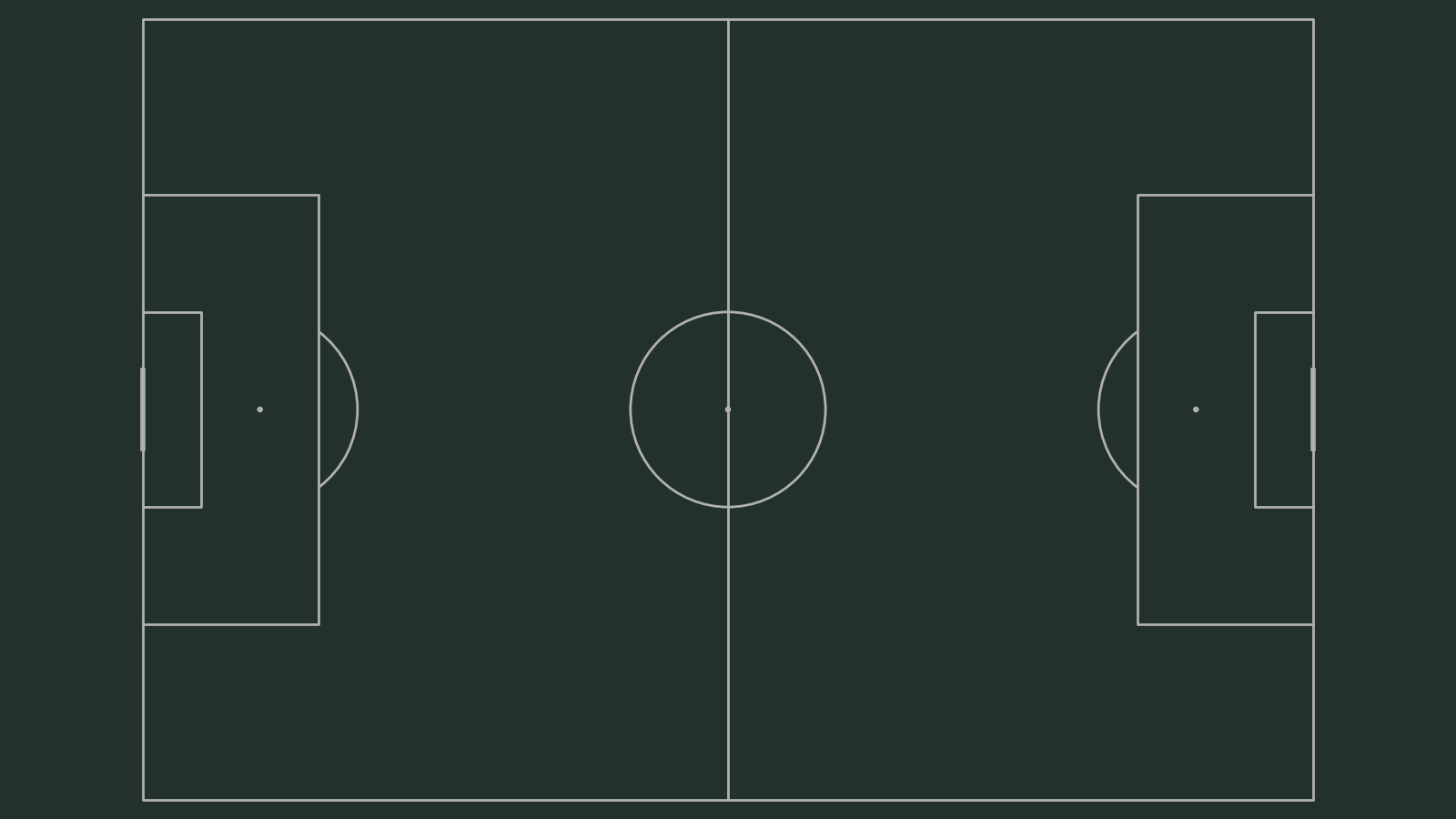## 16 by 9 horizontal grass

Now let’s get the largest pitch possible for a 16:9 figure but with grassy stripes. See Caley Graphics for some inspiration on how you might add titles on the pitch.

```FIGWIDTH = 16
FIGHEIGHT = 9
NROWS = 1
NCOLS = 1
MAX_GRID = 1

# here we setup the padding to get a 16:9 aspect ratio for the axis
# note 80 is the StatsBomb width and 120 is the StatsBomb length
# this will extend the (axis) grassy effect to the figure edges
pitch_color='grass', stripe=True, line_color='white')

# calculate the maximum grid_height/ width
GRID_WIDTH, GRID_HEIGHT = pitch.grid_dimensions(figwidth=FIGWIDTH, figheight=FIGHEIGHT,
nrows=NROWS, ncols=NCOLS,
max_grid=1, space=0)
# plot
fig, ax = pitch.grid(figheight=FIGHEIGHT, grid_width=GRID_WIDTH, grid_height=GRID_HEIGHT,
title_height=0, endnote_height=0)
```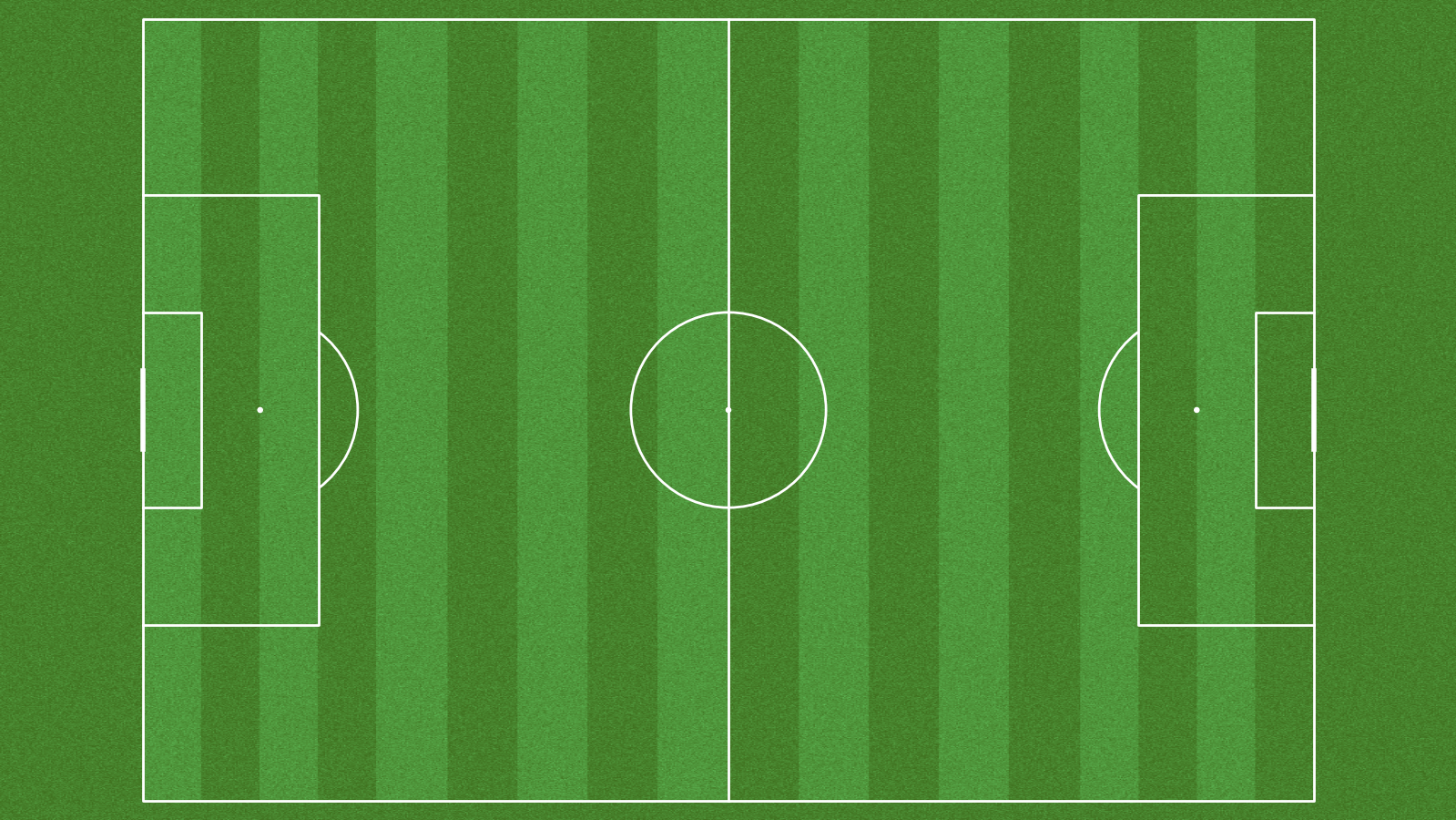## 16 by 9: three vertical pitches

Three vertical pitches fits nicely in the 16:9 aspect ratio. Here we plot with a title and endnote axis too.

```FIGWIDTH = 16
FIGHEIGHT = 9
NROWS = 1
NCOLS = 3
SPACE = 0.09
MAX_GRID = 0.95

pitch_color='grass', stripe=True, line_color='white')

GRID_WIDTH, GRID_HEIGHT = pitch.grid_dimensions(figwidth=FIGWIDTH, figheight=FIGHEIGHT,
nrows=NROWS, ncols=NCOLS,
max_grid=MAX_GRID, space=SPACE)

TITLE_HEIGHT = 0.1
ENDNOTE_HEIGHT = MAX_GRID - (GRID_HEIGHT + TITLE_HEIGHT)

fig, ax = pitch.grid(figheight=FIGHEIGHT, grid_width=GRID_WIDTH, grid_height=GRID_HEIGHT,
space=SPACE, ncols=NCOLS, nrows=NROWS, title_height=TITLE_HEIGHT,
endnote_height=ENDNOTE_HEIGHT, axis=True)
```## 16 by 9: 2 cropped half-pitches

Here we plot two half pitches side-by-side that are cropped so 15 units are taken off each side. This is how I would do game xG comparisons.

```FIGWIDTH = 16
FIGHEIGHT = 9
NROWS = 1
NCOLS = 2
SPACE = 0.05
MAX_GRID = 0.95

pitch_color='grass', stripe=True, line_color='white')

GRID_WIDTH, GRID_HEIGHT = pitch.grid_dimensions(figwidth=FIGWIDTH, figheight=FIGHEIGHT,
nrows=NROWS, ncols=NCOLS,
max_grid=MAX_GRID, space=SPACE)
TITLE_HEIGHT = 0.08
ENDNOTE_HEIGHT = 0.04

fig, ax = pitch.grid(figheight=FIGHEIGHT, grid_width=GRID_WIDTH, grid_height=GRID_HEIGHT,
space=SPACE, ncols=NCOLS, nrows=NROWS, title_height=TITLE_HEIGHT,
endnote_height=ENDNOTE_HEIGHT, axis=True)
```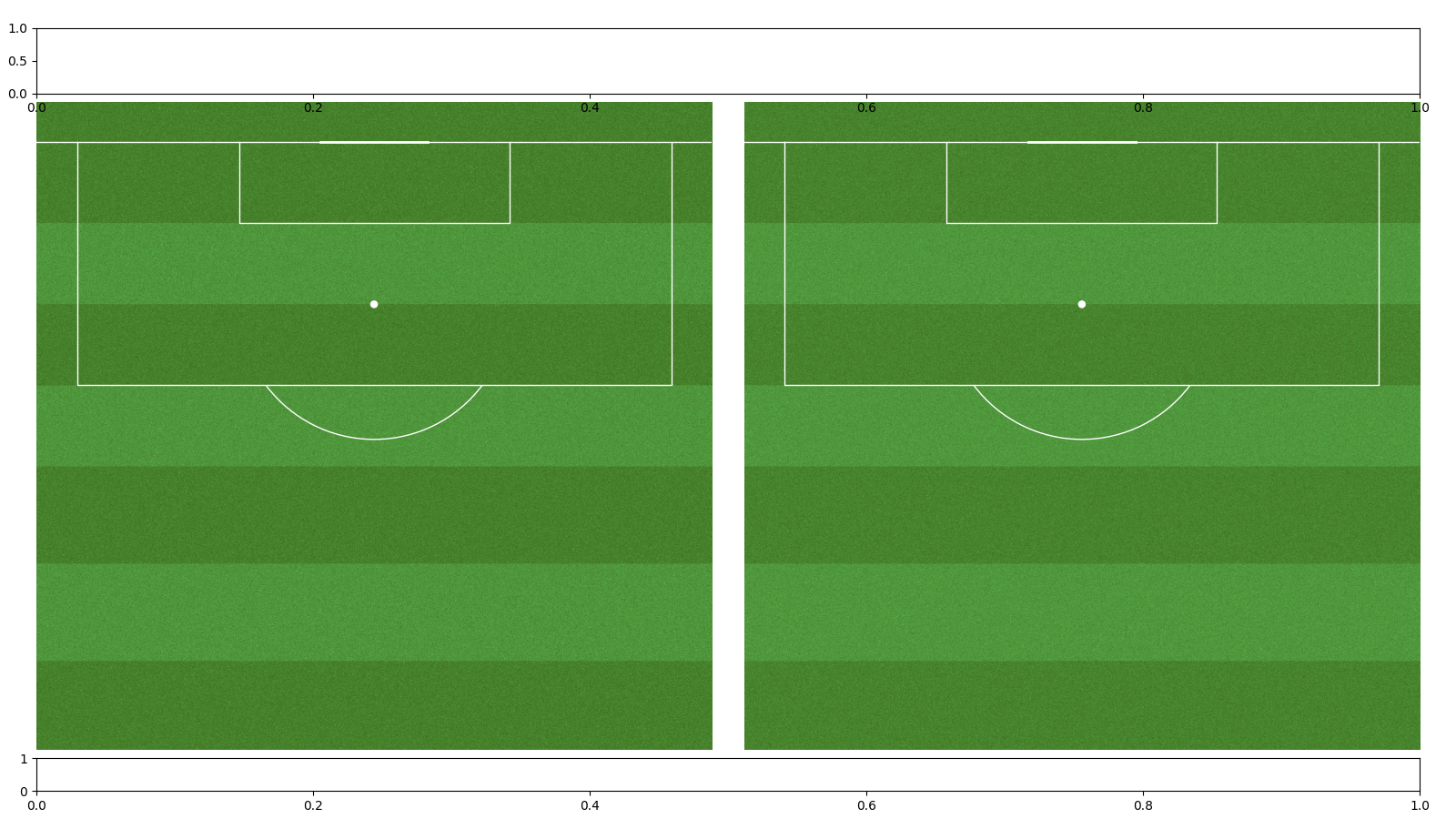## 16 by 9: team of pitches

Here we plot 15 pitches (11 players + 3 subs + 1 pitch for the whole team).

```FIGWIDTH = 16
FIGHEIGHT = 9
NROWS = 3
NCOLS = 5
SPACE = 0.1
MAX_GRID = 0.98

pitch_color='grass', stripe=True, line_color='white')

GRID_WIDTH, GRID_HEIGHT = pitch.grid_dimensions(figwidth=FIGWIDTH, figheight=FIGHEIGHT,
nrows=NROWS, ncols=NCOLS,
max_grid=MAX_GRID, space=SPACE)

TITLE_HEIGHT = 0.15
ENDNOTE_HEIGHT = 0.05

fig, ax = pitch.grid(figheight=FIGHEIGHT, grid_width=GRID_WIDTH,
grid_height=GRID_HEIGHT, space=SPACE,
ncols=NCOLS, nrows=NROWS, title_height=TITLE_HEIGHT,
endnote_height=ENDNOTE_HEIGHT, axis=True)
```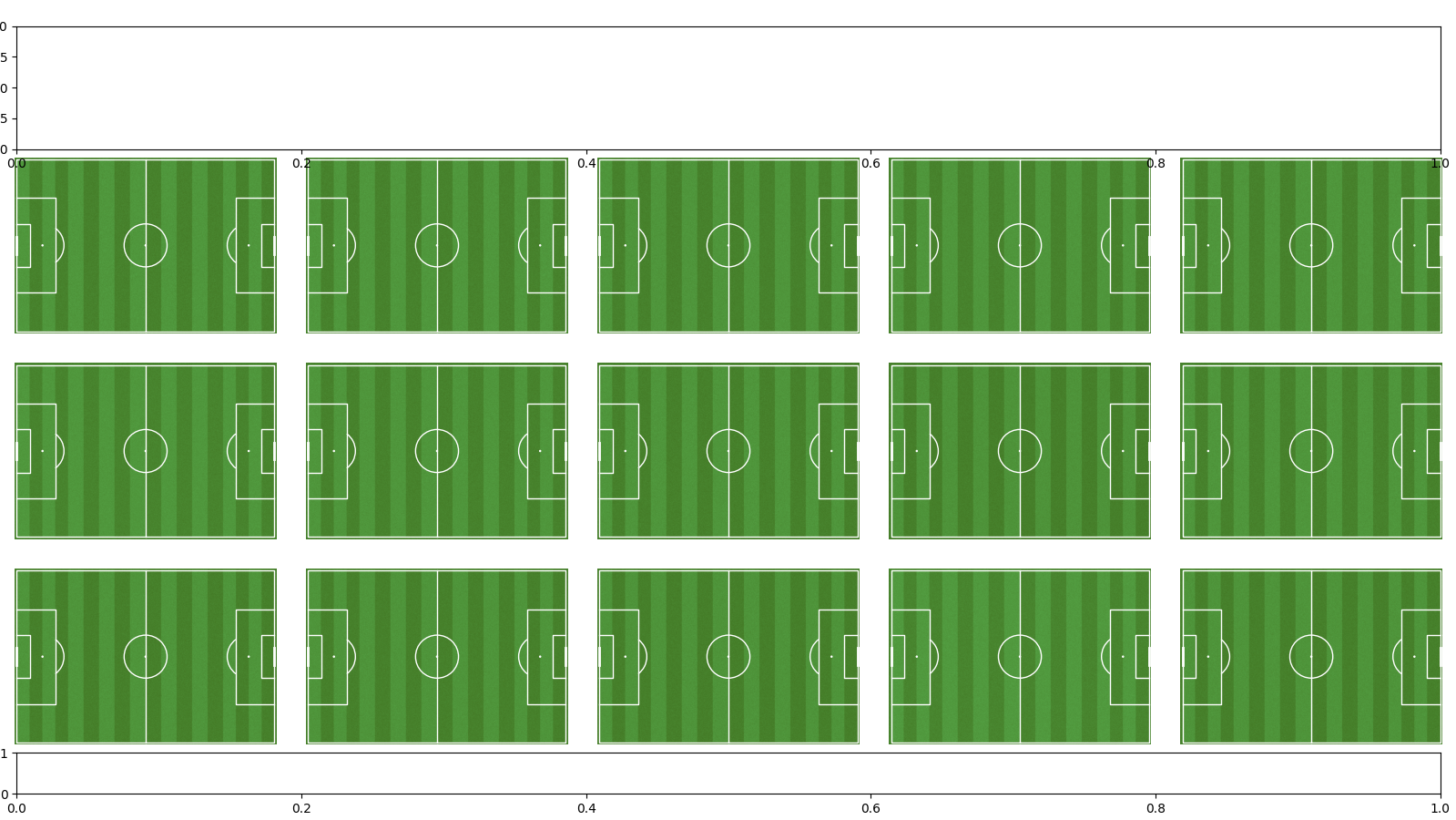## 7 by 8

Most of the Twitter aspect ratios are around 1.5 - 1.8 with the exception of the 7 by 8 aspect ratio. This isn’t a great aspect ratio for pitches, but seems to work okay for one vertical pitch (with a bit of extra space either side).

```FIGWIDTH = 7
FIGHEIGHT = 8
NROWS = 1
NCOLS = 1
SPACE = 0
MAX_GRID = 1

# here we setup the padding to get a 16:9 aspect ratio for the axis
# note 80 is the StatsBomb width and 120 is the StatsBomb length
# this will extend the (axis) grassy effect to the figure edges
pitch_color='grass', stripe=True, line_color='white')

GRID_WIDTH, GRID_HEIGHT = pitch.grid_dimensions(figwidth=FIGWIDTH, figheight=FIGHEIGHT,
nrows=NROWS, ncols=NCOLS,
max_grid=MAX_GRID, space=SPACE)
TITLE_HEIGHT = 0
ENDNOTE_HEIGHT = 0

fig, ax = pitch.grid(figheight=FIGHEIGHT, grid_width=GRID_WIDTH,
grid_height=GRID_HEIGHT, space=SPACE,
ncols=NCOLS, nrows=NROWS, title_height=TITLE_HEIGHT,
endnote_height=ENDNOTE_HEIGHT, axis=True)

plt.show()  # If you are using a Jupyter notebook you do not need this line
```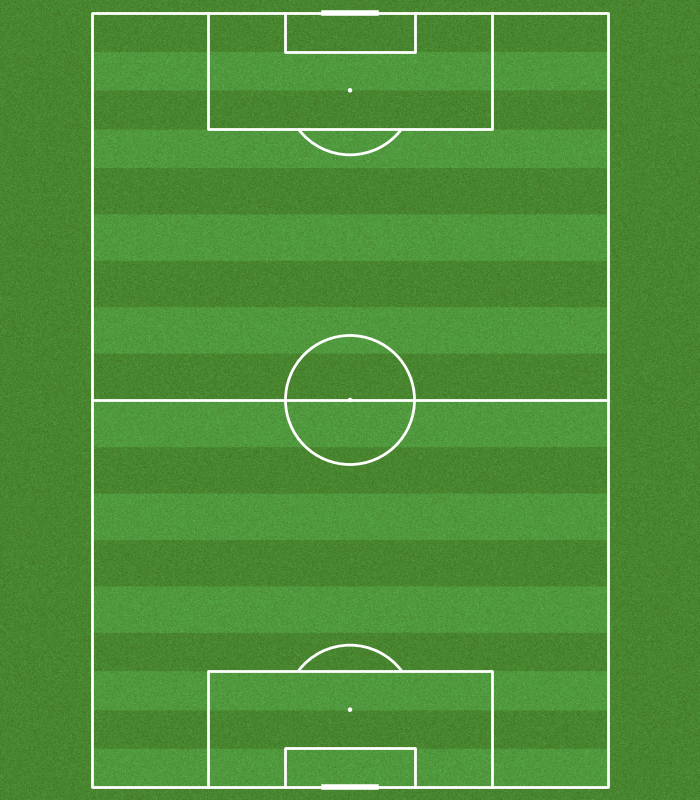Total running time of the script: ( 0 minutes 5.828 seconds)

Gallery generated by Sphinx-Gallery